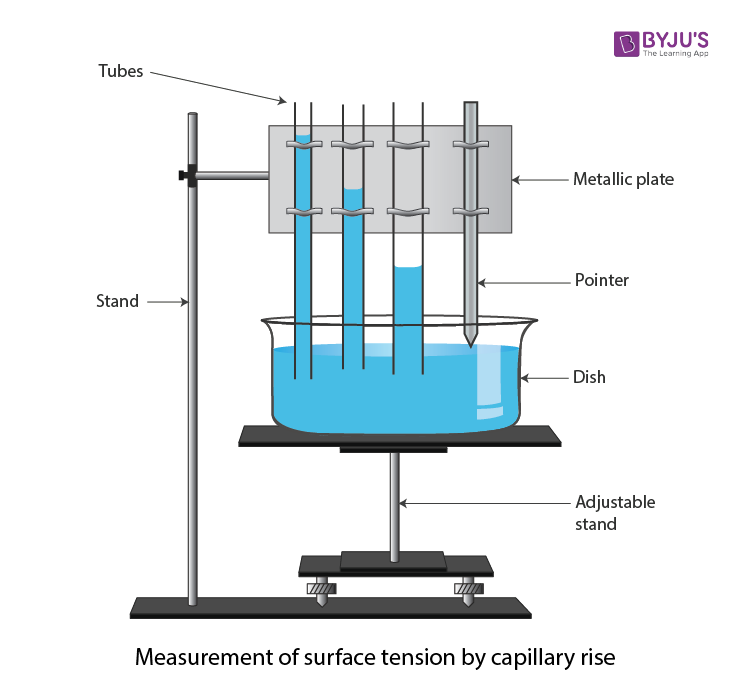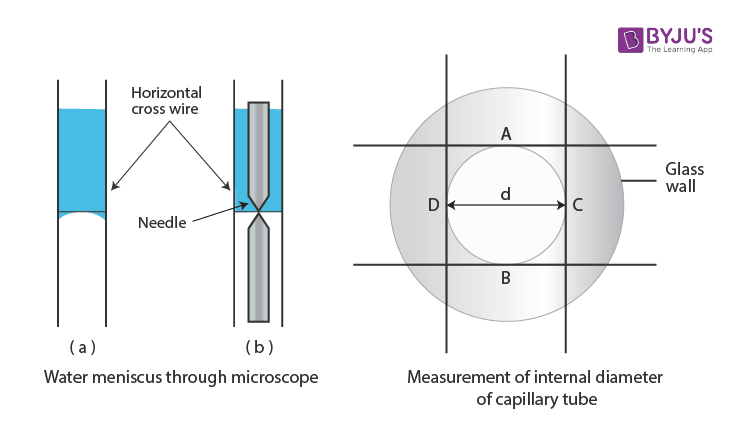# To Determine the Surface Tension of Water by Capillary Rise Method

Surface tension is the tendency of a liquid surface to shrink into the minimum surface area possible. The capillary rise method is one of the techniques to determine the surface tension of a liquid such as water. While experimenting with a capillary tube, it is observed that when a liquid rises in the tube, the weight of the liquid column is supported by the upward force of surface tension acting along the circumference of the points of contact. Let us learn about the experiment and the observations in detail.

## Aim

To find the surface tension of water by capillary rise method.

## Apparatus/ Materials Required

• Three capillary tubes of different radii
• A tipped pointer clamped in a metallic plate with a handle
• Travelling microscope
• A flat bottom open dish
• Thermometer
• Clean water in a beaker
• Clamp and a stand

## Diagram## Theory

The surface tension of water is given by the formula

$$\begin{array}{l}T=\frac{r(r+h/3)\rho g}{2\cos \theta }\end{array}$$

where, r is the radius of cross-section, g is the acceleration due to gravity, ρ is the density of the liquid, h is the capillary rise, θ is the contact angle.

## Procedure

(a) Arranging the apparatus

1. Place the adjustable height stand on the table and make its base horizontal by levelling the screws.
2. Take dirt and grease-free water in an open dish with a flat bottom and put it on top of the stand.
3. Take three capillary tubes of different radii.
4. Clean the tubes and dry them and then clamp them to a metallic plate to increase the radius. Clamp a pointer after the third capillary tube.
5. Clamp the horizontal handle of the metallic plate in a vertical stand so that the capillary tube and the pointer become vertical.
6. Adjust the height of the metallic plate that the capillary tubes dip in the water in the open dish.
7. Adjust the position of the pointer such that the tip touches the water surface.

(b) Measurement of capillary rise

1. Calculate the least count of the travelling microscope for vertical and horizontal scales.
2. Raise the microscope to a suitable height pointed towards the capillary tube with a horizontal axis.
3. Focus the microscope on the first capillary tube.
4. Make the horizontal crosswire touch the central part of the concave meniscus seen convex through the microscope
5. Note the reading of the microscope on the vertical scale.
6. Move the microscope horizontally and bring it in front of the second capillary tube.
7. Lower the microscope and repeat steps 4 and 5
8. Likewise, repeat steps 4 and 4 for the third capillary tube
9. Lower the stand for the pointer tip to be visible.
10. Move the microscope horizontally and bring it in front of the pointer.
11. Lower the microscope and make the horizontal crosswire touch the tip of the pointer.

(c) Measurement of the internal diameter of the capillary tube

1. Place the first capillary tube horizontally on the adjustable stand.
2. Focus the microscope on the end dipped in water. A white circle with a green strip will be visible.
3. Make the horizontal cross-wire touch the inner circle at point A.

## Observation

The least count of the travelling microscope (L.C) = ….. cm.

Height of liquid rise

 S.No Reading of Meniscus Reading of pointer tip Height h1 – h2 = h cm M.S.R N (cm) V.S.R n × LC (cm) Total Reading N + n (LC) h1 (cm) M.S.R N (cm) V.S.R n × LC (cm) Total Reading N + n (LC) h2 (cm) 1 2 3

The internal diameter of the capillary

 S.No Microscope Reading for cross wire in position Internal Diameter Internal Radius d/2 r (cm) (A) (cm) (B) (cm) (C) (cm) (D) (cm) Vertical AB (cm) Horizontal CD (cm) Mean $$\begin{array}{l}\frac{AB+CD}{2}\end{array}$$ d (cm) 1 2 3

## Calculation

Put the value h and r for each capillary tube separately and find the values of T using the following formula:

$$\begin{array}{l}T=\frac{r(h+r/3)\rho g}{2\cos \theta }\end{array}$$

Find the mean value of the obtained T values as follows:

$$\begin{array}{l}T=\frac{T_{1}+T_{2}+T_{3}}{3}\end{array}$$
= _____ dynes cm–1.

## Result

The surface tension of water at t °C is _____ dynes cm–1.

## Viva Voce

1. Explain the relationship between surface tension and surface energy.

Answer: The relationship between surface tension and surface energy is given as follows:

Surface Energy = Surface tension × Change in area

2. Which side of the liquid surface has more pressure?

Answer: The pressure is more on the concave side of the free liquid surface.

3. What is capillary?

Answer: An open-ended tube with a fine bore is known as capillary.

4. Why should the liquid be free from grease?

Answer: Grease reduces the surface tension of the liquid.

5. What is the surface tension of water?

Answer: The surface tension of water is 7.275 × 10–2 N-m–1 at 20 °C.

6. Why do you measure the internal diameter of the capillary tube in two mutually perpendicular directions?

Answer: It is done to take the mean to eliminate the error if the bore is not circular.

Stay tuned with BYJU’S to get the latest notification on CBSE along with CBSE syllabus, sample papers, marking scheme and more.

Test Your Knowledge On To Find The Surface Tension Of Water By Capillary Rise Method!